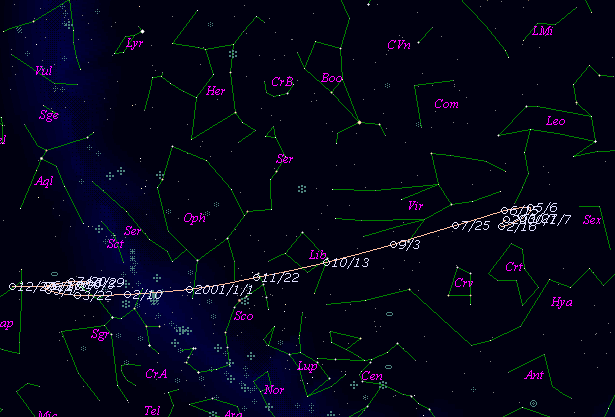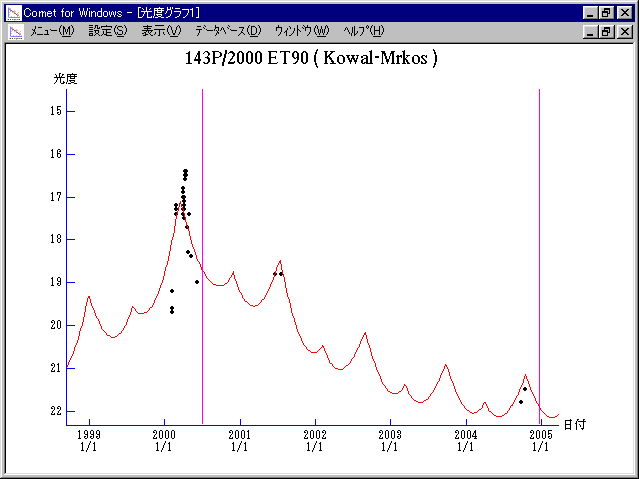# \$B%3%o%k(B-\$B%`%k%3%9WB@1(B

143P/Kowal-Mrkos (2000)###\$B%W%m%U%#!<%k(B

 \$BId9f(B 143P/2000 ET90 \$B:FH/8+F|(B 2000\$BG/(B3\$B7n(B9\$BF|(B \$B:FH/8+8wEY(B 18.9\$BEy(B \$B:FH/8+ Lincoln Laboratory Near-Earth Asteroid Research project

###\$B50F;MWAG(B

```   The following improved orbital elements by Kenji Muraoka, are
from 69 observations 1984 to 2000, perturbations by 9 Plantes,
Moon and 5 minor planets were taken into account.
The mean residual is +/- 0.67 arc seconds.

Epoch  =  2000 Jun  = 2451720.5
T  =  2000 July  1.78795       +/- 0.00166 (m.e.) TT
Peri. =  320.58931                +/- 0.00095
Node  =  245.49591                +/- 0.00158   (2000.0)
Incl. =    4.68385                +/- 0.00012
q  =    2.5468225              +/- 0.0000132 AU
e  =    0.4090186              +/- 0.0000031
a  =    4.3094799              +/- 0.0000074 AU
n  =    0.11017087             +/- 0.00000028
P  =    8.946                  +/- 0.0000231  years
```

###\$B@1?^(B###\$B8wEYJQ2=(B

```        H = 13.6  G = 0.15
```##### \$B50F;MWAG\$OB<2,7r<#;a\$N7W;;\$K\$h\$k\$b\$N\$G\$9!#(B \$B@1?^\$O(B StellaNavigator Ver.2.0 for Windows (\$B%"%9%H%m%"!<%D(B \$BJTCx(B / \$B%"%9%-!<=PHG6I4)(B) \$B\$G:n@.\$7\$?\$b\$N\$G\$9!#(B \$B8wEY%0%i%U\$O(BComet for Windows\$B\$G:n@.\$7\$?\$b\$N\$G\$9!#(B